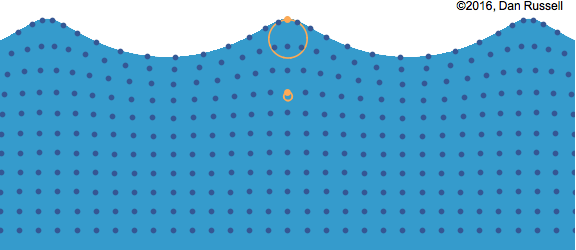It looks like you're using Internet Explorer 11 or older. This website works best with modern browsers such as the latest versions of Chrome, Firefox, Safari, and Edge. If you continue with this browser, you may see unexpected results.

# Physics 9: Waves

Waves

Cambridge

Assignments

## Theory

ReflectionAngle of incidence = Angle of reflection

Refraction

Refraction is the change in direction of a wave when it transmits from one medium to another.The angle of incidence and the angle of refraction can be determined by Snell’s law given by the following formulawhere n1 and n2 are the refractive indexes for their respective mediumsFast-to-slow: towards normal; slow-to-fast: away from normal

In addition, the refractive index n1 and n2 are related by the following equationwhere v1 and v2 are the speed of the waves in their respective mediums and λ1 and λ2 are the wavelength of the waves of their respective mediumsTotal Internal ReflectionThe refractive index and the critical angle are related by the following equationTotal internal reflection only occurs when the light ray propagates from a optically denser medium to an optically less dense medium.

Diffraction

When waves pass through a gap in a barrier, they spread out into space beyond the barrier. This spreading of the waves is called diffraction.The waves are diffracted the most when the width of the gap is similar to the wavelength of the waves.

(The effect is strongest in c and weakest in a)• ### Amplitude, wavelength, frequency, period and wave speed

 Amplitude Period (T) Frequency (f) Maximum displacement of the oscillating object Time taken for one complete oscillation (in seconds) Number of times the object oscillates per unit time (usually one second) f=1/T###Wave speed can be calculated by the following equation• ### Transverse and longitudinal waves

 Transverse wave Longitudinal wave The direction of oscillation is perpendicular to the direction of the energy transferThe direction of oscillation is parallel to the direction of the energy transferExample:      Water waves    Wave in a string flicked up and down    Light (electromagnetic waves) Example:      Wave produced in a spring    Sound waves    Earthquake P-waves

### FYI

 Transverse wave Longitudinal wave A point with maximum positive displacement is called a crest.   A point with minimum displacement is called a trough. A region where particles are closed to each other is called a compression.   A region where particles are furthest apart from each other is called a rarefaction.• ### The nature of electromagnetic waves

All EM waves travel in vacuum at the same speed of 3*10^8m/s.

EM waves are transverse waves.• ### The nature of sound waves

The speed of sound in 20 degrees Celsius dry air is approximately 343.2m/s.

Sound waves are longitudinal waves.## Visuals

Gap is large compared to wavelength                                                       Gap is small compared to wavelengthExplanation                                                                         In glass fiber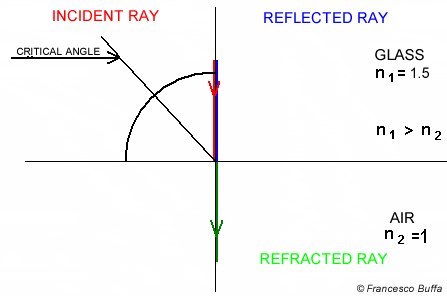In water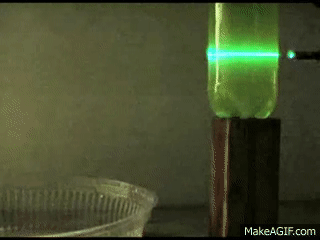Dispersion of light through a prismAngle of incidence is not 90°                                                     Angle of incidence is 90°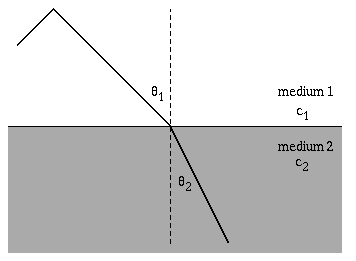Longitudinal Waves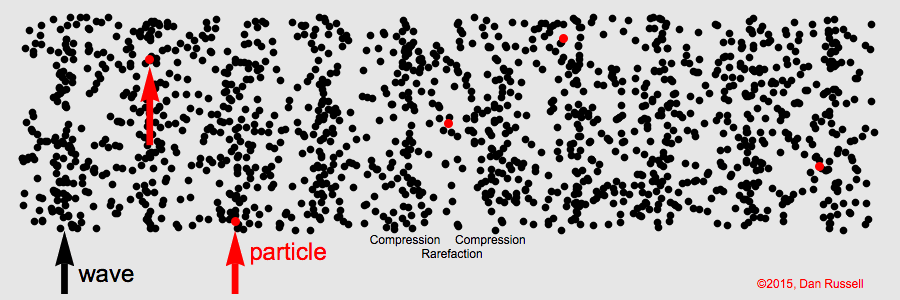Transverse waves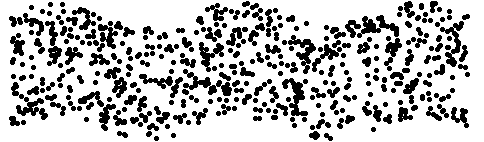Water waves